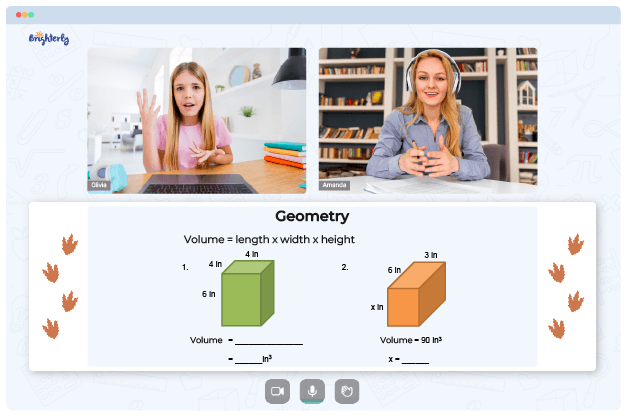# Perimeter Of A Rectangle Worksheets

Kids must learn how to calculate the figures around a rectangle as they progress in their grades. A perimeter of a rectangle is defined as the distance around it. Kids can learn to make these calculations using a rectangle worksheet’s perimeter.

## Benefits of the perimeter of rectangle worksheet

There are certain benefits to using the perimeter of squares and rectangles worksheet to teach children. Here are some of these benefits:

### It helps the kids retain formulas

For kids to solve the perimeter of a rectangle, they need to use specific formulas. These formulas help them follow a path to finding the solution to the problems involved in this math concept. The perimeter of a rectangles worksheet teaches them repeatedly practice until they can recognize them by heart.

Math for Kids

Is Your Child Struggling With Math?
1:1 Online Math Tutoring### It helps the kid’s scale difficulty levels easier

Educators designed the perimeter of a rectangle worksheets 4th grade with different difficulty levels that they have to cross with each solution they reach. This pattern makes it easy for the kids to write texts and exams.### Perimeter Of A Rectangle Worksheets PDF

Perimeter Of A Rectangle Worksheet### Perimeter Of A Rectangle Worksheets PDF

Perimeter Of Squares And Rectangles Worksheet### Perimeter Of A Rectangle Worksheets PDF

Perimeter Of Rectangle Worksheet### Perimeter Of A Rectangle Worksheets PDF

Perimeter Of A Rectangle Worksheets 4Th Grade

Troubles with Geometry?• Does your child struggle to grasp the concept of geometry?
• Try lessons with an online tutor.

Is your child having difficulties with understanding geometry basics? An online tutor could provide the necessary support.